# RD Sharma Solutions Class 8 Chapter 3 Squares and Square Roots Exercise 3.5

Here you can get free RD Sharma Solutions for Class 8 Maths Chapter 3 Squares and Square Roots Exercise 3.5. All RD Sharma Book Solutions are given here exercise wise for the chapter Squares and Square Roots. RD Sharma Solutions are helpful in the preparation of several school level, graduate and undergraduate level competitive exams. Practicing questions from RD Sharma Mathematics Solutions for Class 8 Chapter 3 Squares and Square Roots is proven to enhance your math skills.

 Class: Class 8th Chapter: Chapter 3 Exercise: Exercise 3.5 Name: Squares and Square Roots

## RD Sharma Solutions Class 8 Chapter 3 Squares and Square Roots Exercise 3.5

RD Sharma Class 8 Solutions Chapter 3 for Squares and Square Roots Exercise 3.5 are given below.

RD Sharma Solutions Class 8 Chapter 3 Squares and Square Roots Exercise 3.5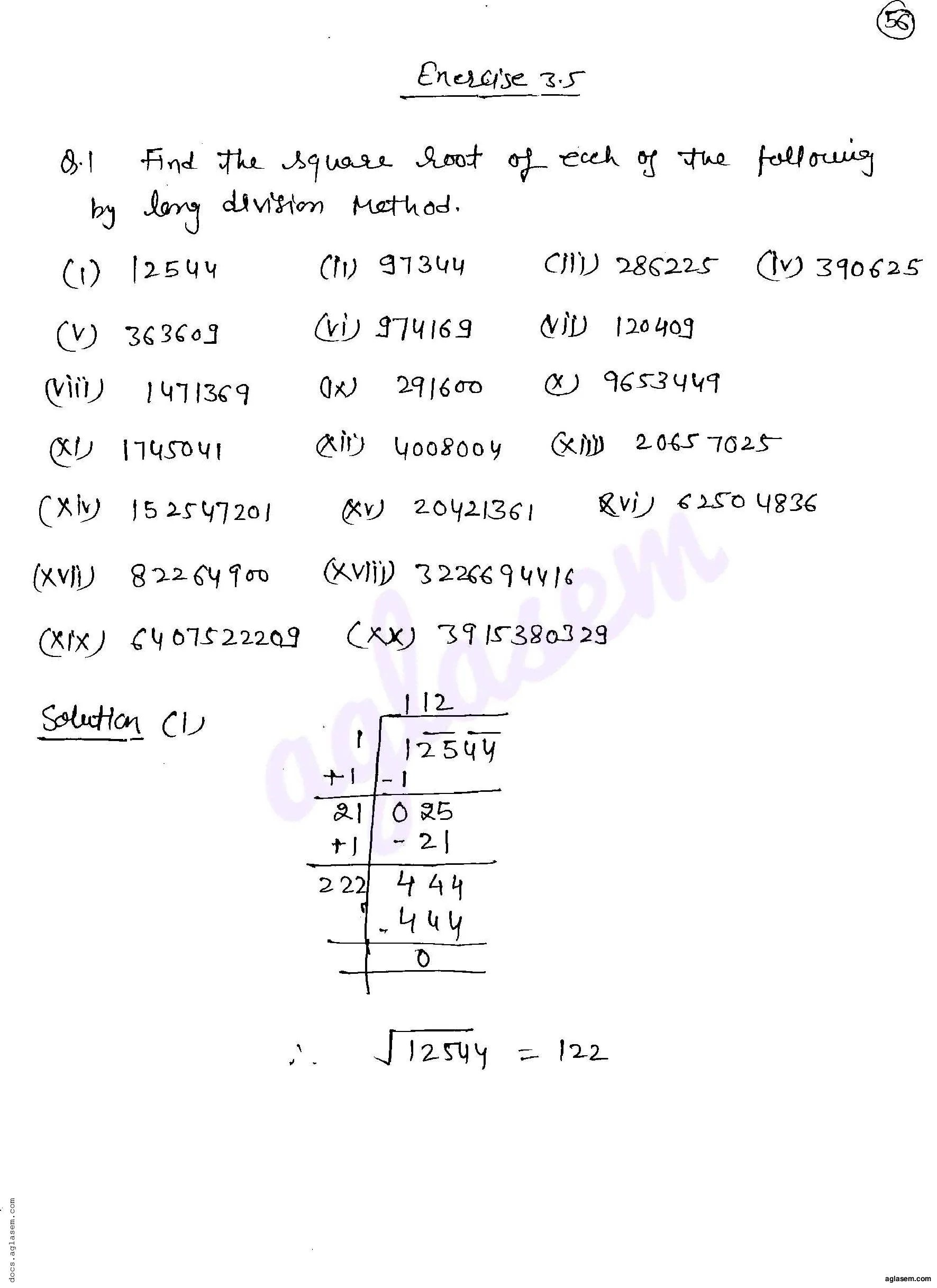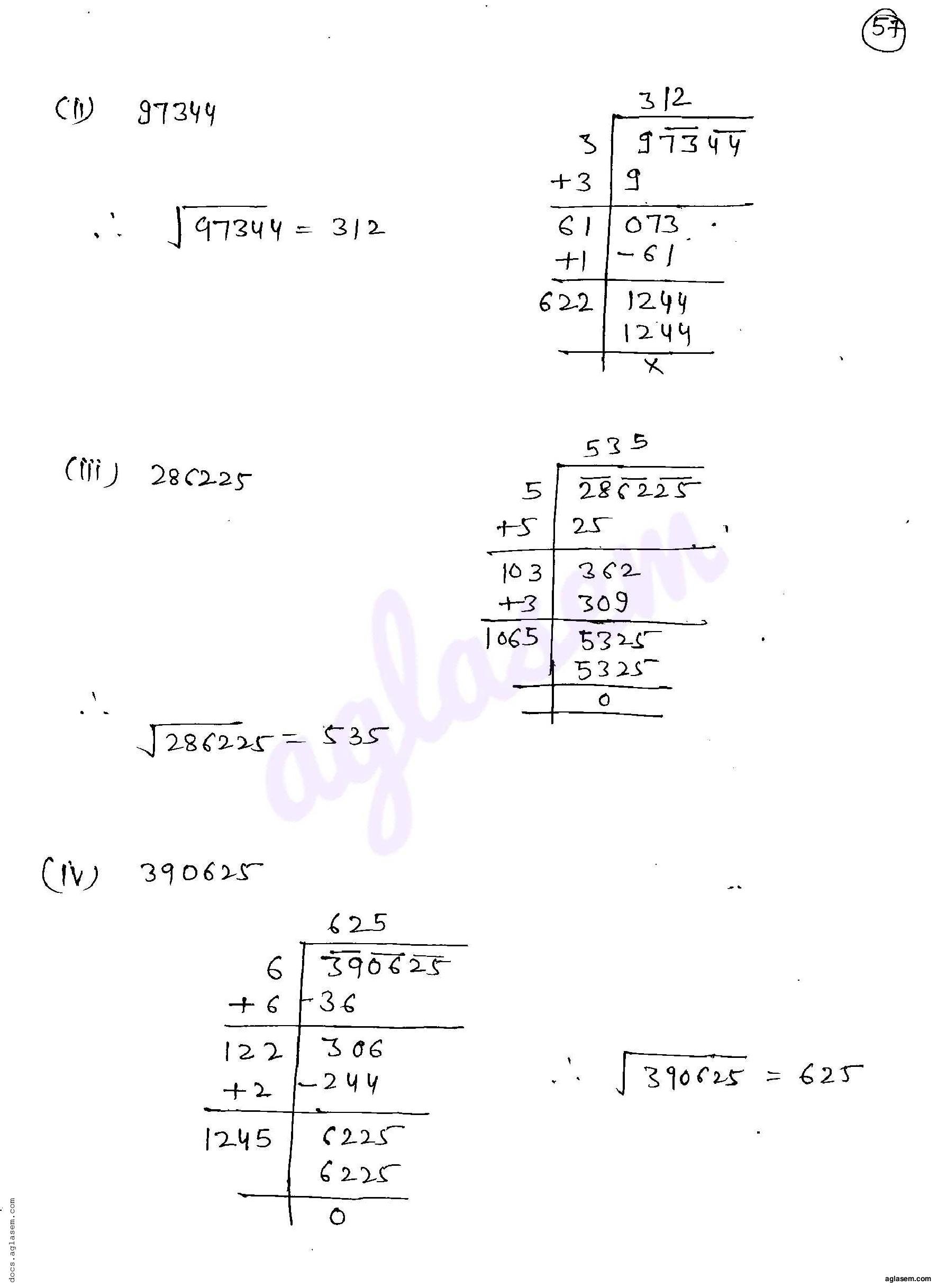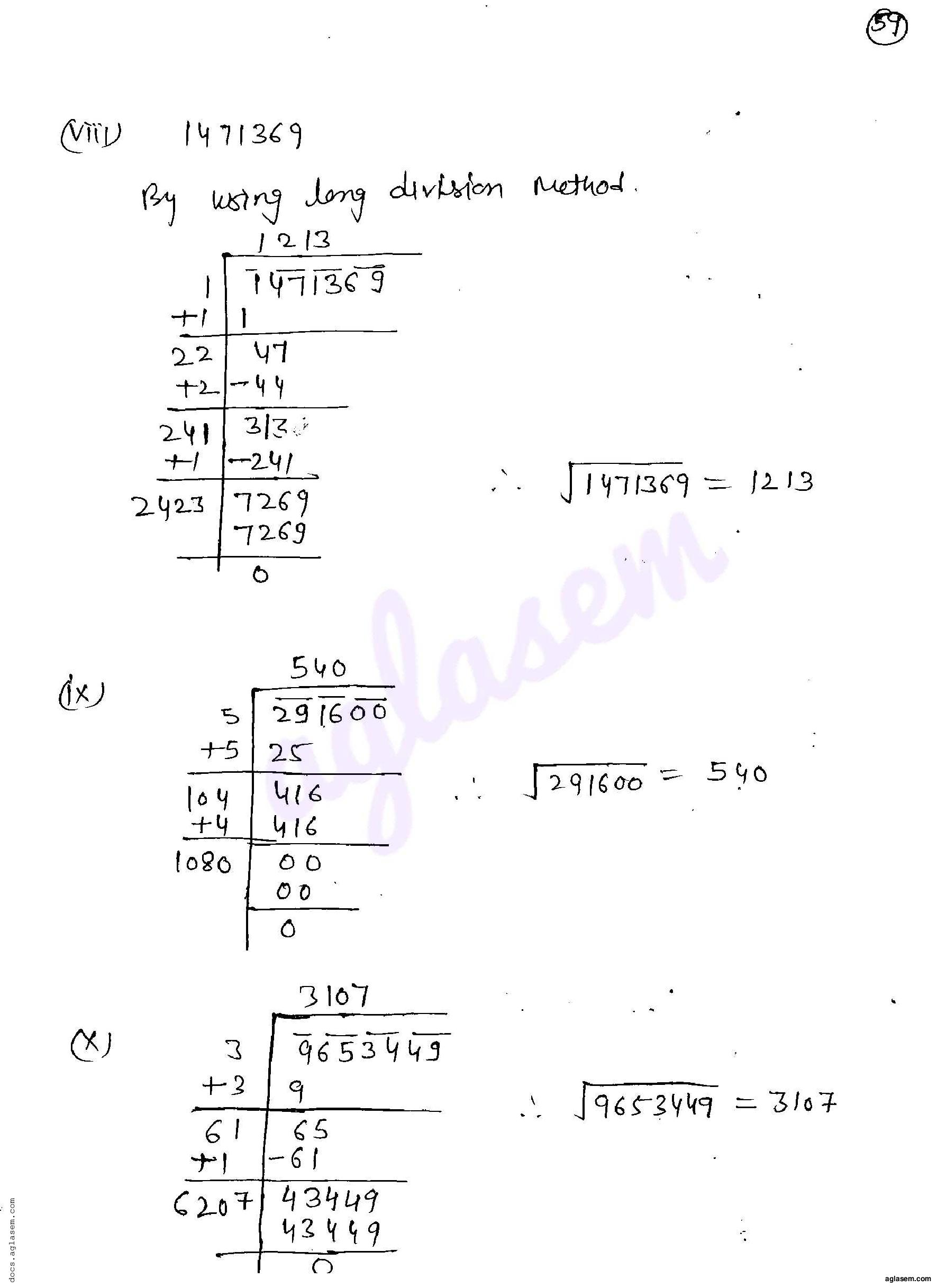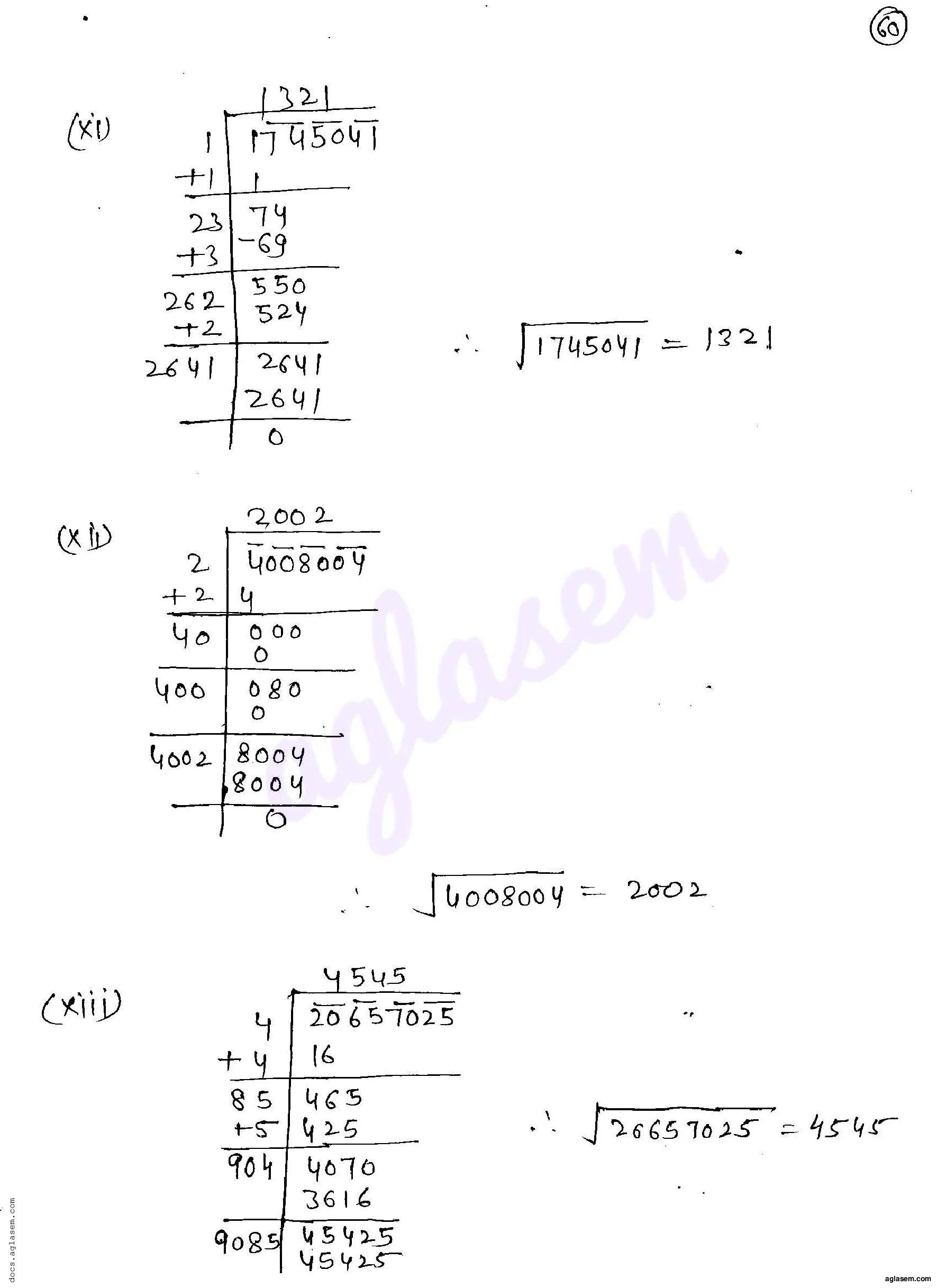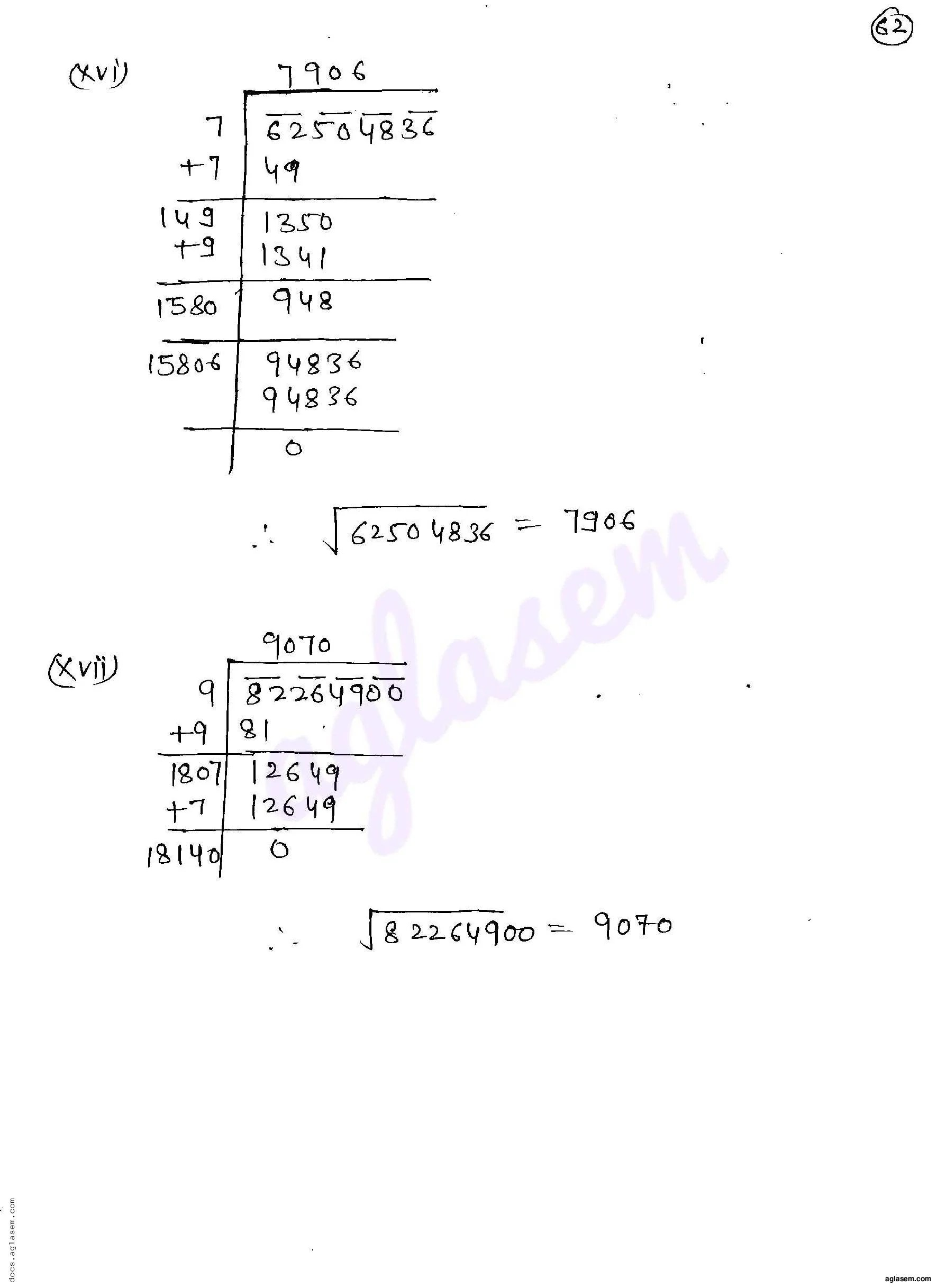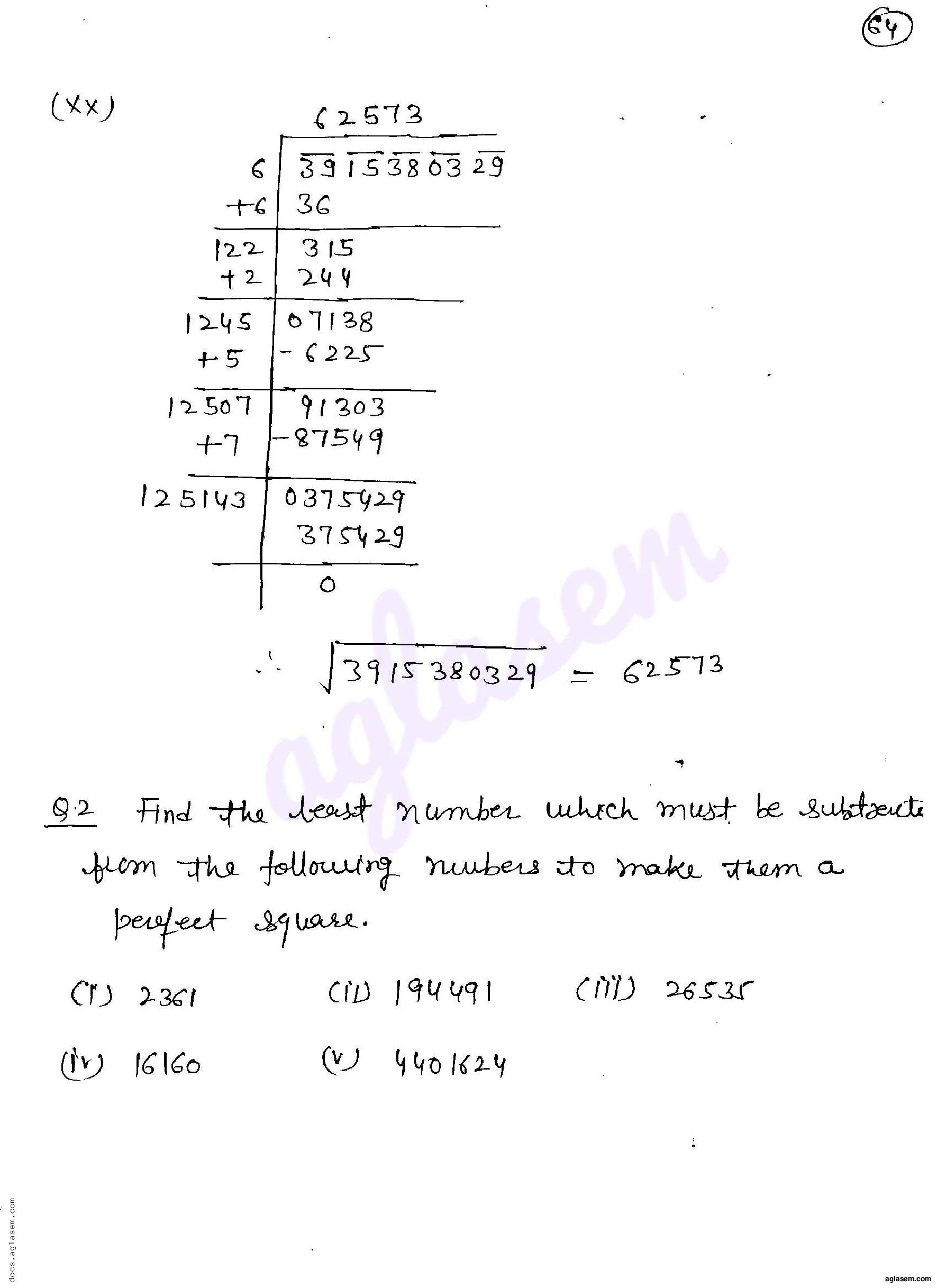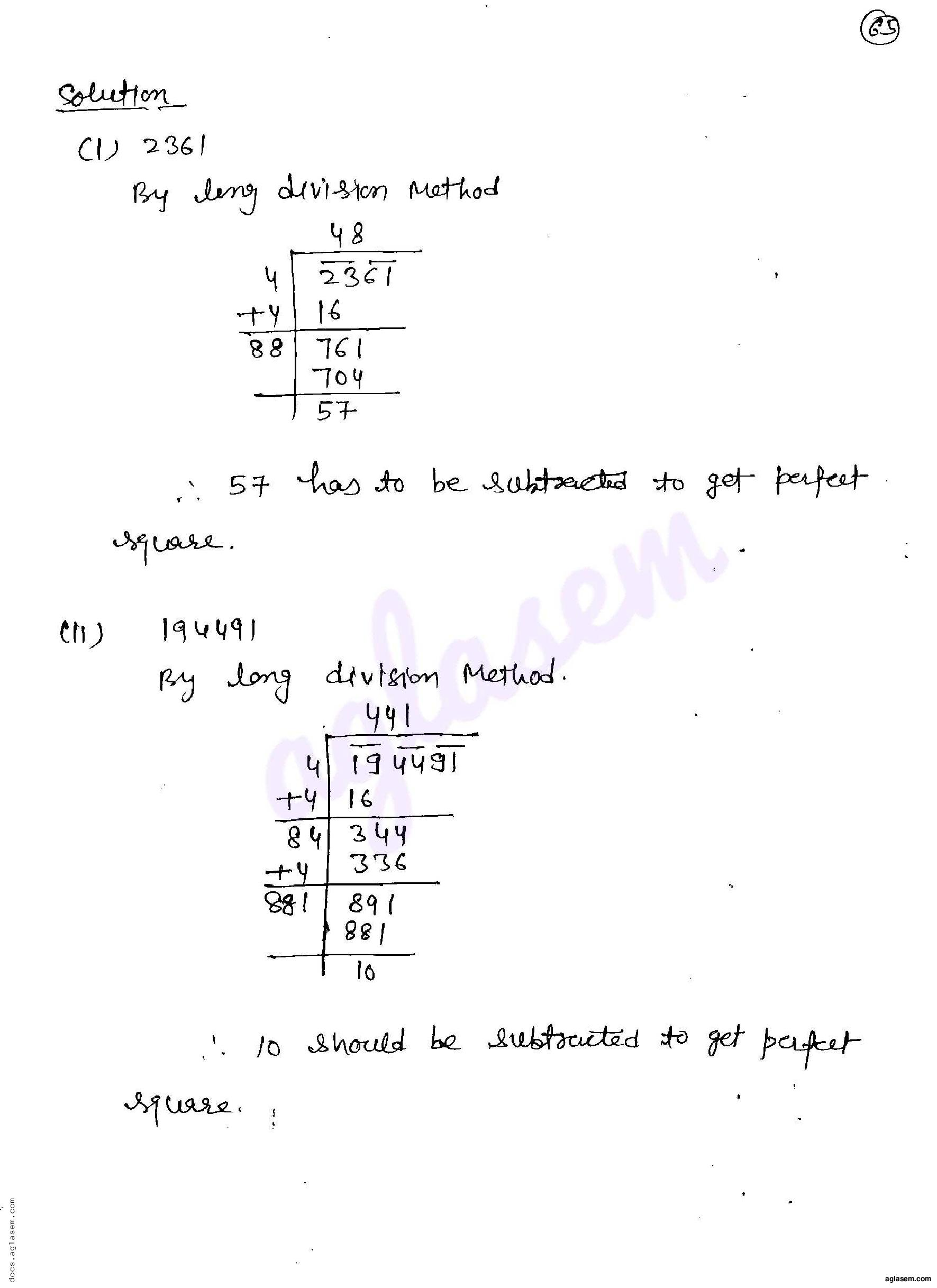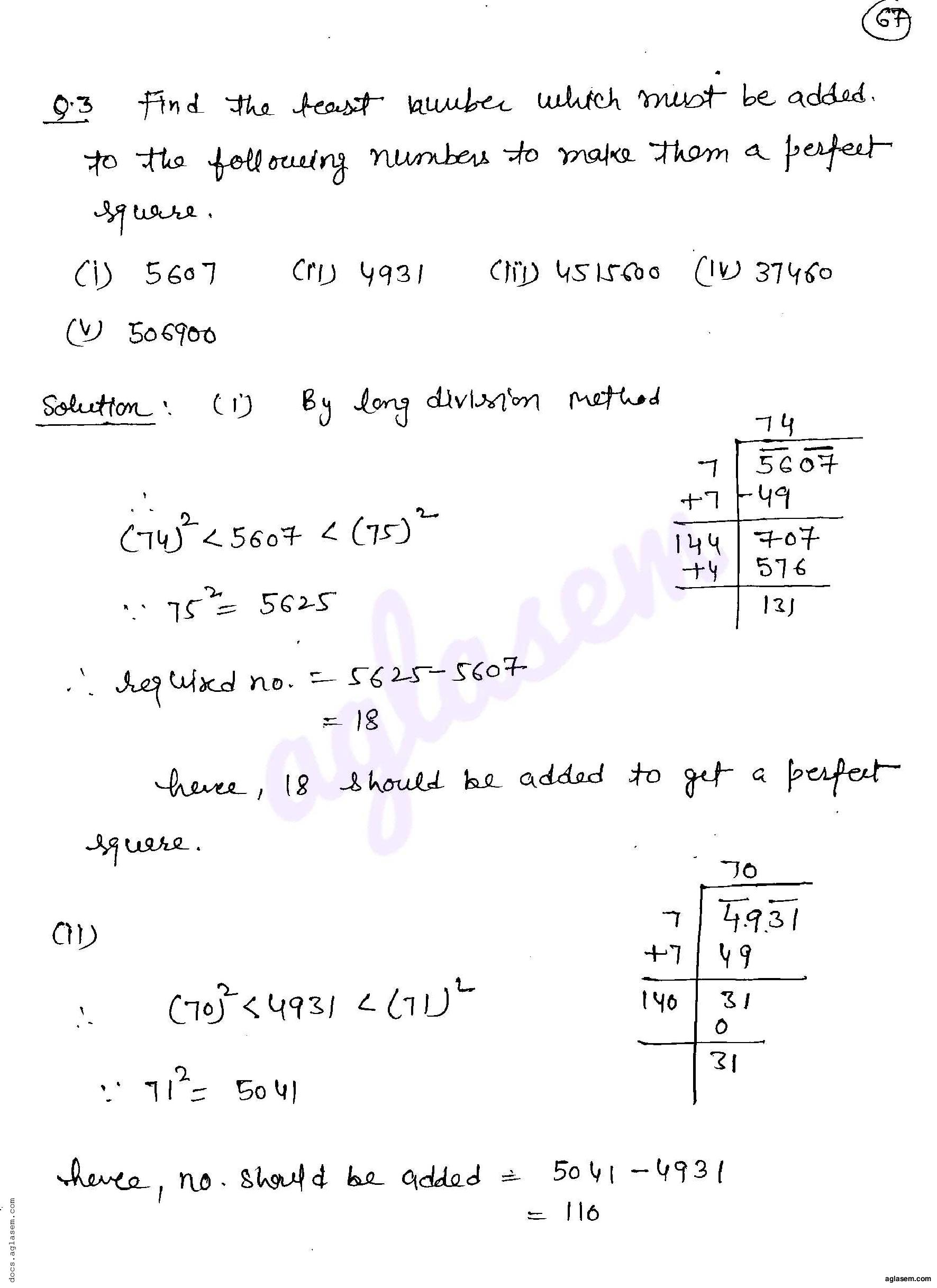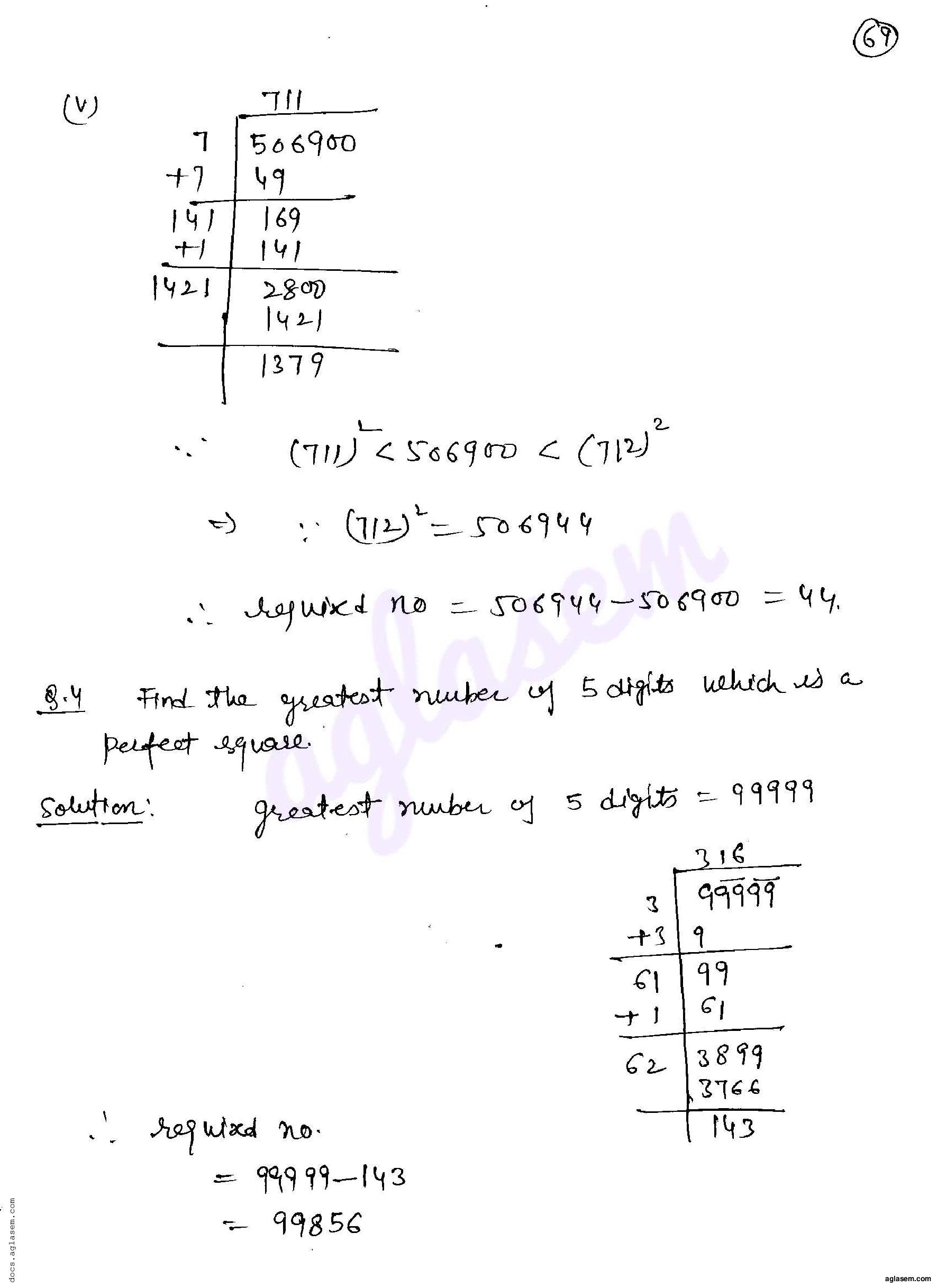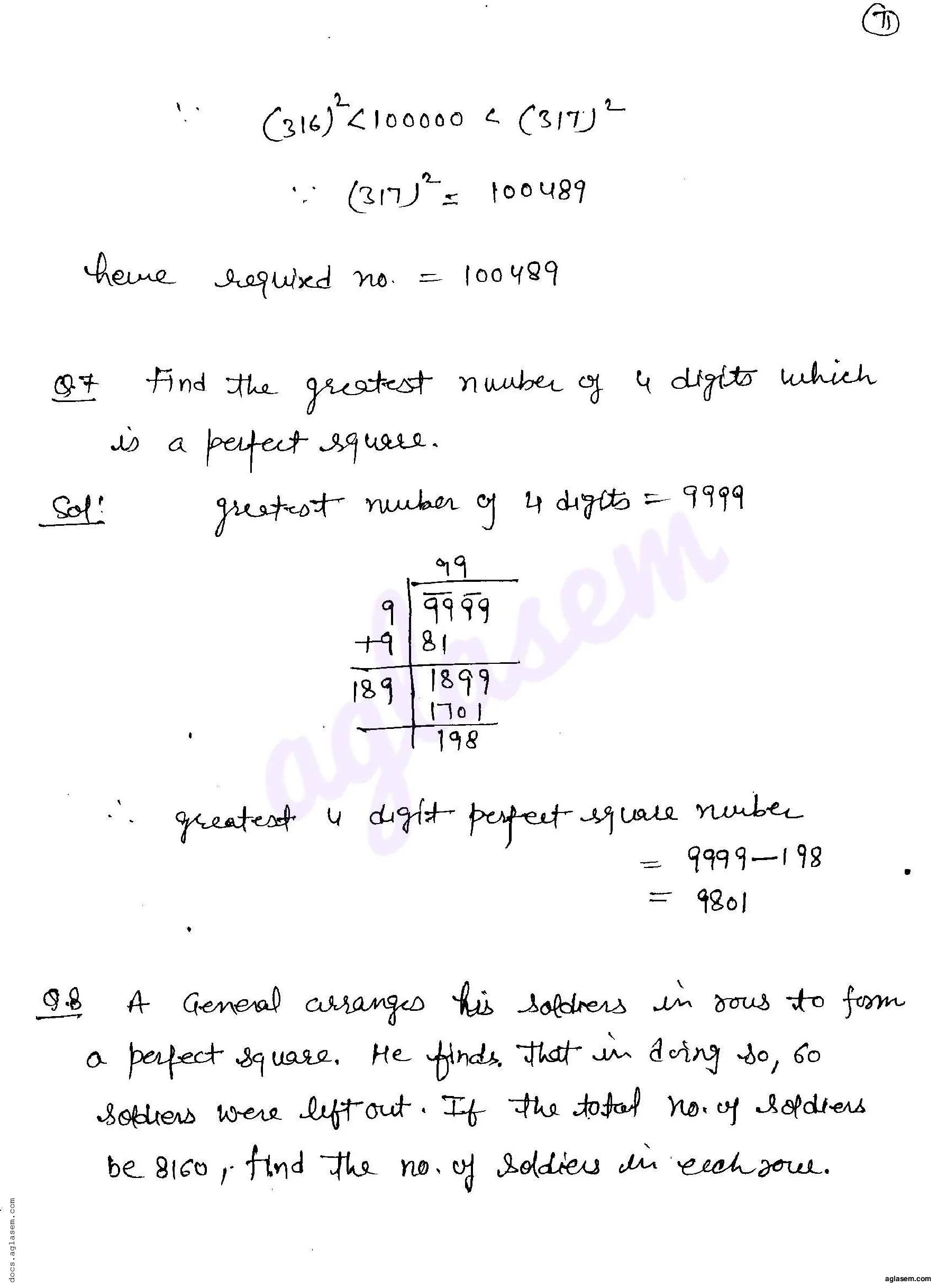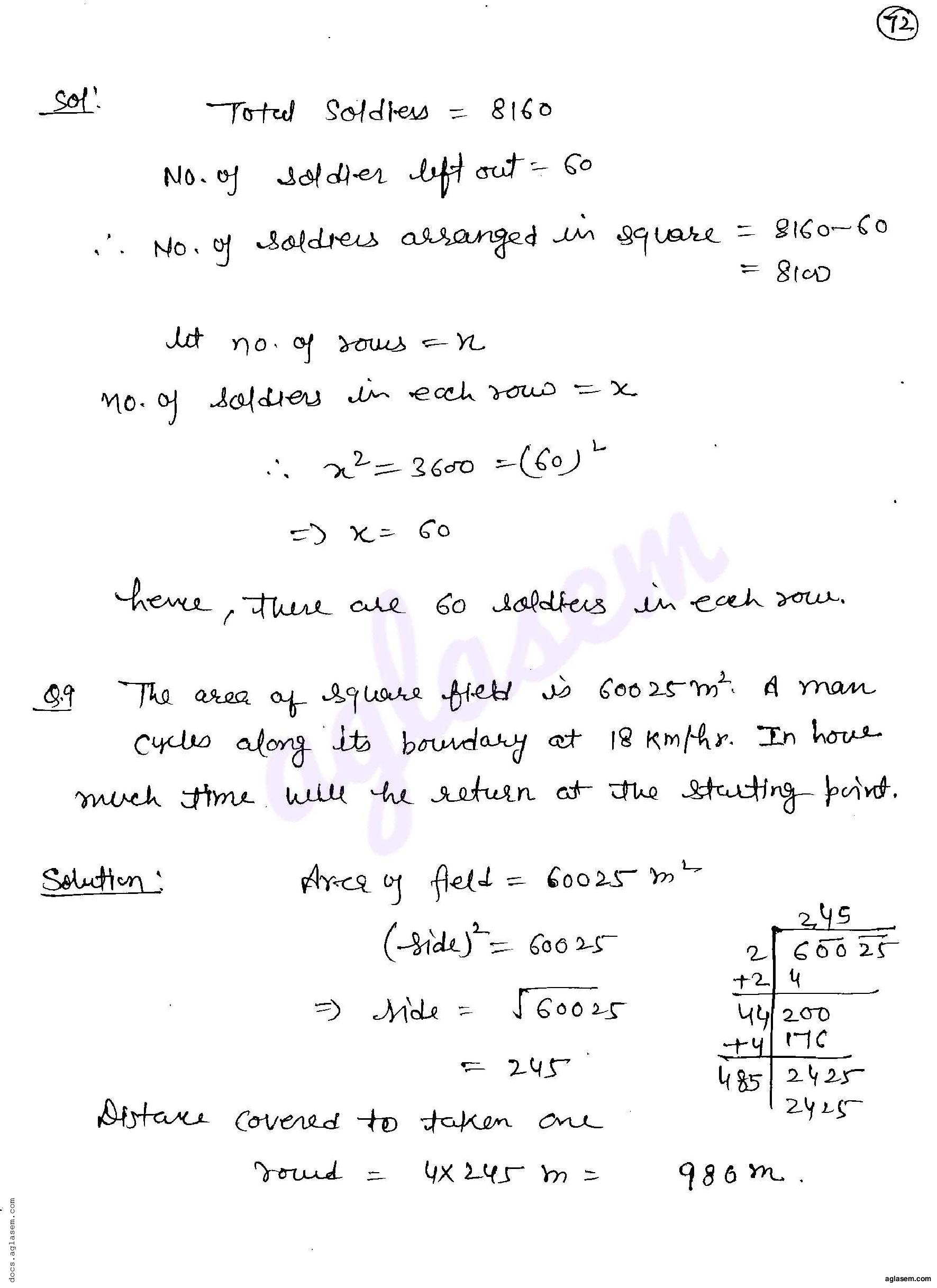RD Sharma Solutions for Class 8 Maths PDF download for Chapter 3 Squares and Square Roots Exercise 3.5 is available here for free.

 « Previous Next »

### RD Sharma Solutions Class 8 Chapter 3 Exercise Wise

You can also see RD Sharma Solutions of all exercises of Chapter 3 – Squares and Square Roots from here.

### RD Sharma Solutions Class 8 Chapter Wise

If you want RD Sharma solutions of any topic other than Chapter 3 Squares and Square Roots, check it from here. Mathematics by RD Sharma has all chapters that are in your Class 8 syllabus.

Click to rate this post!
[Total: 2 Average: 5]